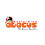### An Integrated Look at the Meaning of Integers and Rationals

"The mathematics of the elementary and middle school curriculum is not trivial, and the underlying concepts and structures are worthy of serious, sustained study by teachers."

Access to the linear presentation of Integer and Rational number construction is provided through       a link at the end of this post.

## The Difference Concept

I am going to get ahead of myself here to take a crack at meaning and interpretation. At this stage you can take it as conjecture based on the feeling that whatever turns out will make a sense we might have anticipated. Active learning is not just following an argument, it is more like provoking one, even with yourself.

Let's not worry about number systems yet and assume "numbers" can do what we ask of them. We know two numbers can be either added or multiplied.  And we know that one number can be different from another. We are talking about a mathematical difference; how can arithmetic turn one number into another, how can it come up with a value for the "difference".

We have two operations to work with: addition and multiplication. For many of us it is not a stretch to see addition of an "additive difference"( call it "AD") making up the difference from b(3) to a(7).
additive difference:   b→a = a - b
7 = 3 + 4
The additive difference "turns b into a": it adds a and subtracts b. It could be negative, but leave that aside here.

What about multiplication? Why might we think there could be a "multiplicative difference"(MD)? Unless we know there is, it could only be intuition; something like: if it is possible it is likely. Is it possible? What would it look like? Simply interchange the "+" with "x":
multiplicative difference?   b→a
a = b x MD

The multiplicative difference "turns b into a": it divides by b and multiplies by a. It is a "rational number".
multiplicative difference:   b→a = a/b
a = b x MD.
MD = a/b.

7 = 3 x (7/3)

We have found two "differences" between two numbers; one additive and the other multiplicative. These two exhaust the possibilities; each leads to one of the two number systems beyond Natural Numbers in School arithmetic: additive to Integers and  multiplicative to Rationals.

I like to think of an additive difference as a translator, and a multiplicative difference as a transformer. Integers translate, Rationals transform. You might prefer "augmenter" or "extender" to "translator", and "changer" or "shape-shifter" to "transformer".  The name is not the thing; the thing is a relational sense for meaning, not a definitional one.

Try thinking about the meaning of rational numbers in these terms of what they do instead of the usual "ratios" or "proportions"  for what they "are". The former is demonstrated, the latter asserted.

We can now turn to the other side of an active approach to arithmetic, the constructive one where an object "is" what goes into its architecture and construction, not the label put on it:

Constructing Number System for School Arithmetic

1.